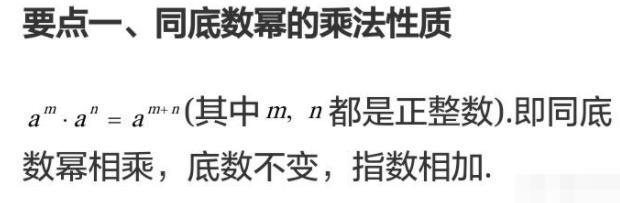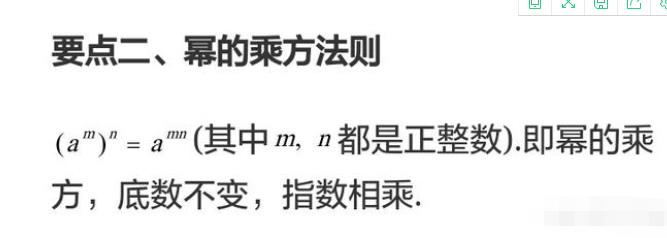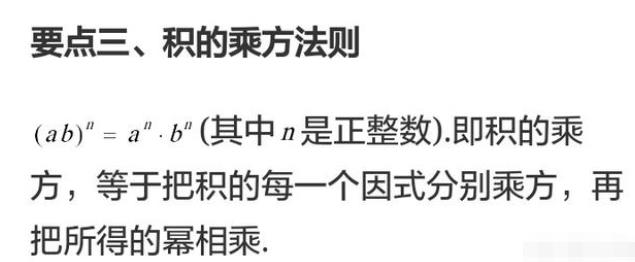# 若3*9的m次方乘以27的m次方=3的21次方，则m应该是多少？

若3*9的m次方乘以27的m次方=3的21次方，则m应该是多少?很容易看的出来，这是考查幂指数方程，解答幂指数方程得时候，套用公式，然后进行一些计算。同学们先要将上文字的问题理解清楚，题目中说若3*9的m次方乘以27的m次方=3的21次方，等价于3 x 9^m x 27^m = 3^21，我们需要解决的就是这个等式，求出m的值是多少?

先把数学算式列出来如下：

3 x 9^m x 27^m = 3^21 ;

(9^m：表示9的m次方，后面一样)

转化成同底数幂乘积的形式：

9^m=(3^2)^m=3^(2m);

27^m=(3^3)^m=3^(3m);

原式即为：

3 x 3^(2m)x 3^(3m)=3^21;

即：

3^(1+2m+3m)= 3^21;

所以：

1+2m+3m = 21 ;

解得：

m = 4以上是若3*9的m次方乘以27的m次方=3的21次方，则m应该是多少该题的解题思路和步骤，希望对咱们学生了解幂指数这类型的问题有一定的帮助。